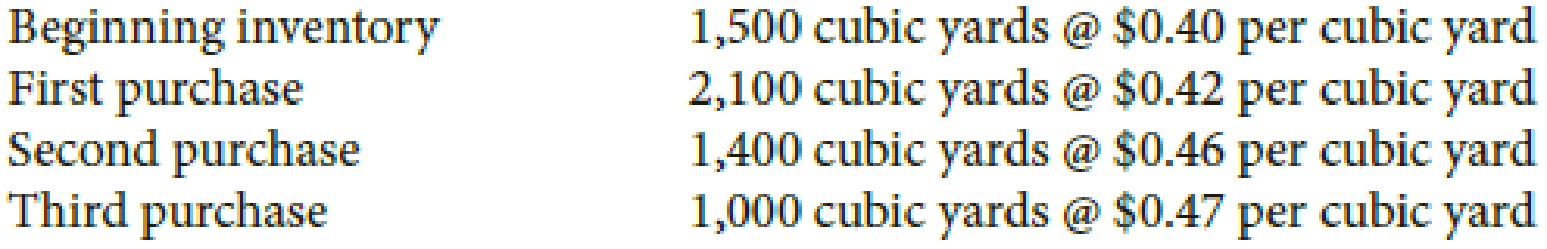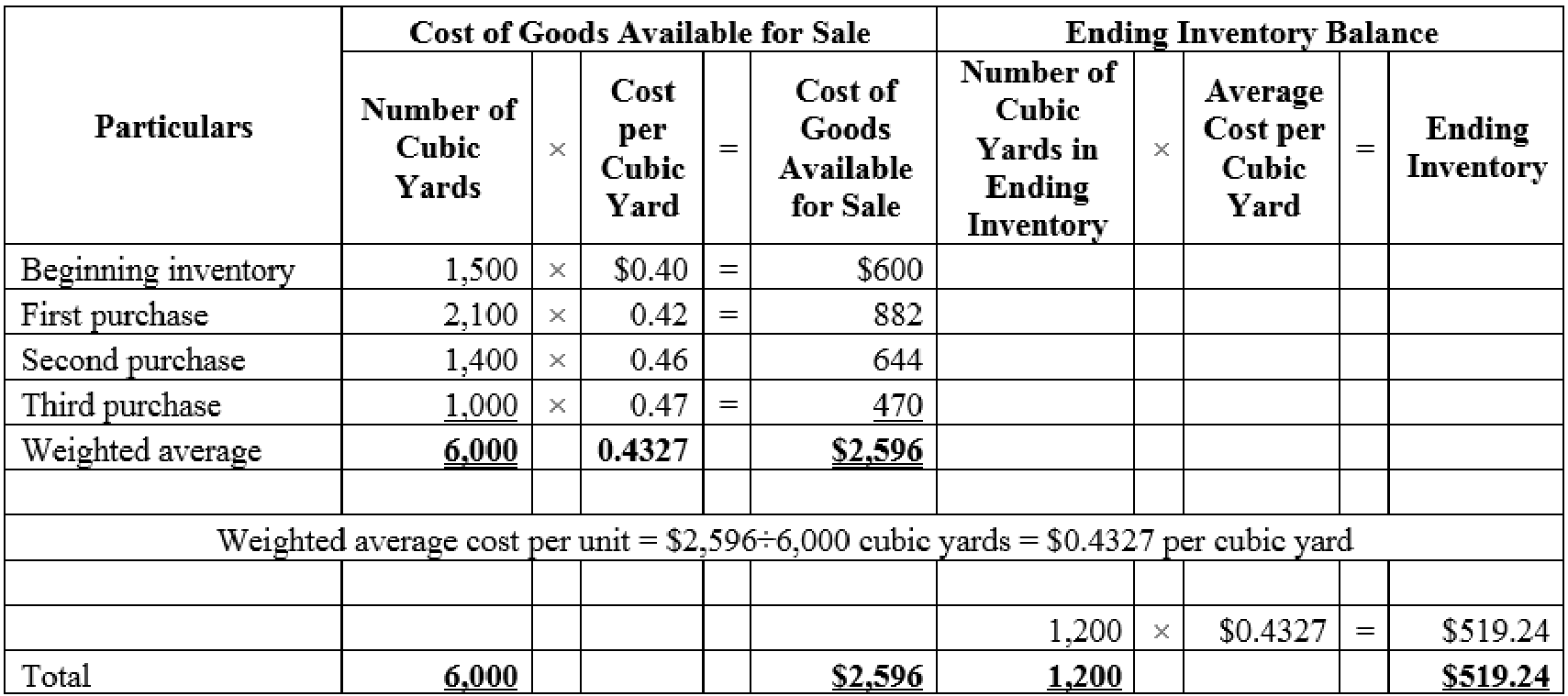Chapter C, Problem 1P

Chapter
Section
Textbook Problem

Bean Nursery sells bark to its customers at retail. Bean buys bark from a plywood mill in bulk and transports the bark in its own trucks. Information relating to the beginning inventory and purchases of bark is as follows:RequiredFind the cost of 1,200 cubic yards in the ending inventory by the weighted-average-cost method. Carry average cost per cubic yard to four decimals.Check FigureCost of ending inventory, \$519.24

To determine

Compute the cost of ending inventory using weighted-average-cost method.

Explanation

Weighted-average cost method: Under average cost method, company calculates a new average after every purchase. It is determined by dividing the cost of goods available for sale by the units on hand.

Compute the cost of ending inventory of 1,200 cubic yards using weighted-average-cost method.Table (1)

Conclusion

Thus, the cost of 1,200 cubic yards using weighted-average-cost is \$519.24.

The Solution to Your Study Problems

Bartleby provides explanations to thousands of textbook problems written by our experts, many with advanced degrees!

Get Started

Chapter C Solutions

Find more solutions based on key concepts